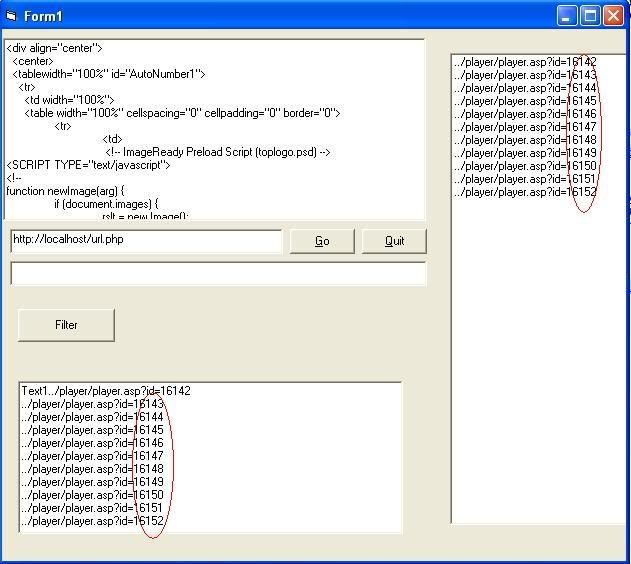# Thread: How to construct a URL from dynamic id values?

1. ## How to construct a URL from dynamic id values?

Hi all:

I have this code that outputs Id= values like this :

../player/player.asp?id=16142
../player/player.asp?id=16143
.....
.....
.....

The output is displayed in textbox and listbox and i want to keep them . All i want add another text box to
currently project that outputs the constructed URL for all "id = " values . The url looks like this with value of id in it:

Code:
`http://www.mysite/player/player.asp?sender=java&Id=16142&Id=16143&Id=16144&Id=16145`

The bold parts are id values which are dynamic and it is already produced by the application. i want
use this in url structure.I be happy if u guys help me with it.Thankscode:

Code:
```Private Sub Command1_Click(Index As Integer)

Select Case Index
Case 0:
If txtURL.Text <> "" Then
RichTextBox1.Text = Inet1.OpenURL(txtURL.Text, icString)
End If

Case 1:
End
End Select
End Sub

Private Sub Command2_Click()
Dim sResult() As String, N As Long

If GetLine(RichTextBox1.Text, "../player/player.asp?id=", "')", sResult) Then
' Occurances were found and have been placed in the array
' we'll just Debug.Print them
'For N = LBound(sResult) To UBound(sResult)
'    Debug.Print sResult(N)
'Next N

For N = LBound(sResult) To UBound(sResult)
Text1.Text = Text1.Text & sResult(N) & vbCrLf

Next N

Else
' No occurances were found
End If
End Sub

Private Function GetLine(ByVal sText As String, ByVal sStart As String, ByVal sEnd As String, ByRef sArr() As String) As Boolean
Dim lPos As Long, lEnd As Long, lCount As Long, sTemp() As String

ReDim sTemp(100)

lPos = InStr(1, sText, sStart, vbTextCompare)
Do While lPos
lEnd = InStr(lPos, sText, sEnd, vbTextCompare)
If lEnd Then
'Remove & sEnd from the below line.
'sTemp(lCount) = Mid\$(sText, lPos, lEnd - lPos) & sEnd
sTemp(lCount) = Mid\$(sText, lPos, lEnd - lPos)
lPos = InStr(lEnd, sText, sStart, vbTextCompare)
Else
sTemp(lCount) = Mid\$(sText, lPos)
lPos = 0
End If
lCount = lCount + 1
If lCount > UBound(sTemp) Then ReDim Preserve sTemp(100 + lCount)
Loop

If lCount > 0 Then
ReDim Preserve sTemp(lCount - 1)
sArr = sTemp
End If
GetLine = lCount
End Function```Reply With Quote

2. ## Re: How to construct a URL from dynamic id values?

so u want to take the list of player id's
and make it into one URL like u showed?Reply With Quote

3. ## Re: How to construct a URL from dynamic id values?Originally Posted by Static
so u want to take the list of player id's
and make it into one URL like u showed?
Thank u for u reply. YEs exactly but i do not know how to strip the output out and make that url and put it in textbox for further maniuplation. I be happy if u help me.ThanksReply With Quote

4. ## Re: How to construct a URL from dynamic id values?

this should get u rolling..
NewText will contain the URL with all the ID's in it
VB Code:
```Dim tmp() As String
Dim IDS() As String
Dim NewText As String
tmp = Split(text1,vbCrLf)
NewText = "http://www.mysite/player/player.asp?sender=java&Id="
For x = 0 To UBound(tmp)
IDS = Split(tmp(x),"=")
If IsNumeric(IDS(1)) Then
newtext = newtext & "&Id=" & IDS(1)
End If
Next```Reply With Quote

5. ## Re: How to construct a URL from dynamic id values?Originally Posted by Static
this should get u rolling..
NewText will contain the URL with all the ID's in it
VB Code:
```Dim tmp() As String
Dim IDS() As String
Dim NewText As String
tmp = Split(text1,vbCrLf)
NewText = "http://www.mysite/player/player.asp?sender=java&Id="
For x = 0 To UBound(tmp)
IDS = Split(tmp(x),"=")
If IsNumeric(IDS(1)) Then
newtext = newtext & "&Id=" & IDS(1)
End If
Next```
Thanks for u code. could u tell me where should i place your code ? i want to make the output and constructed the url on click of filter button and textbox name is NewText. I tried the following and it did not work and give me error could u help me fix this ?ThanksTHe bold part is the added code:

Code:
```Private Sub Command2_Click()
Dim sResult() As String, N As Long

If GetLine(RichTextBox1.Text, "../player/player.asp?id=", "')", sResult) Then
' Occurances were found and have been placed in the array
' we'll just Debug.Print them
'For N = LBound(sResult) To UBound(sResult)
'    Debug.Print sResult(N)
'Next N

For N = LBound(sResult) To UBound(sResult)
Text1.Text = Text1.Text & sResult(N) & vbCrLf

Next N

Dim tmp() As String
Dim IDS() As String
Dim NewText As String
tmp = Split(Text1, vbCrLf)
NewText = "http://www.mysite/player/player.asp?sender=java&Id="
For x = 0 To UBound(tmp)
IDS = Split(tmp(x), "=")
If IsNumeric(IDS(1)) Then
NewText = NewText & "&Id=" & IDS(1)
End If
Next

' end of making url code

Else
' No occurances were found
End If
End Sub```Reply With Quote

####Posting Permissions

• You may not post new threads
• You may not post replies
• You may not post attachments
• You may not edit your posts
•